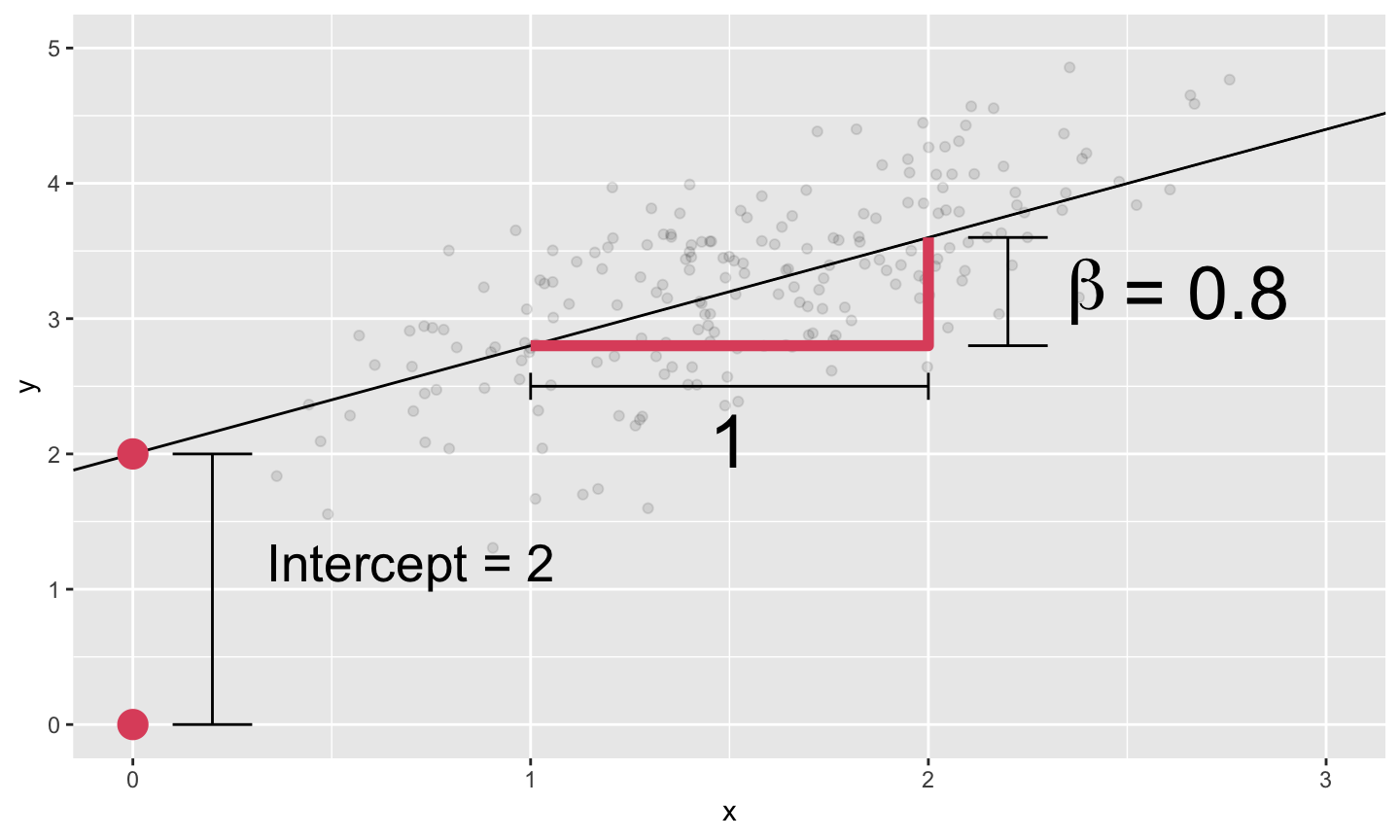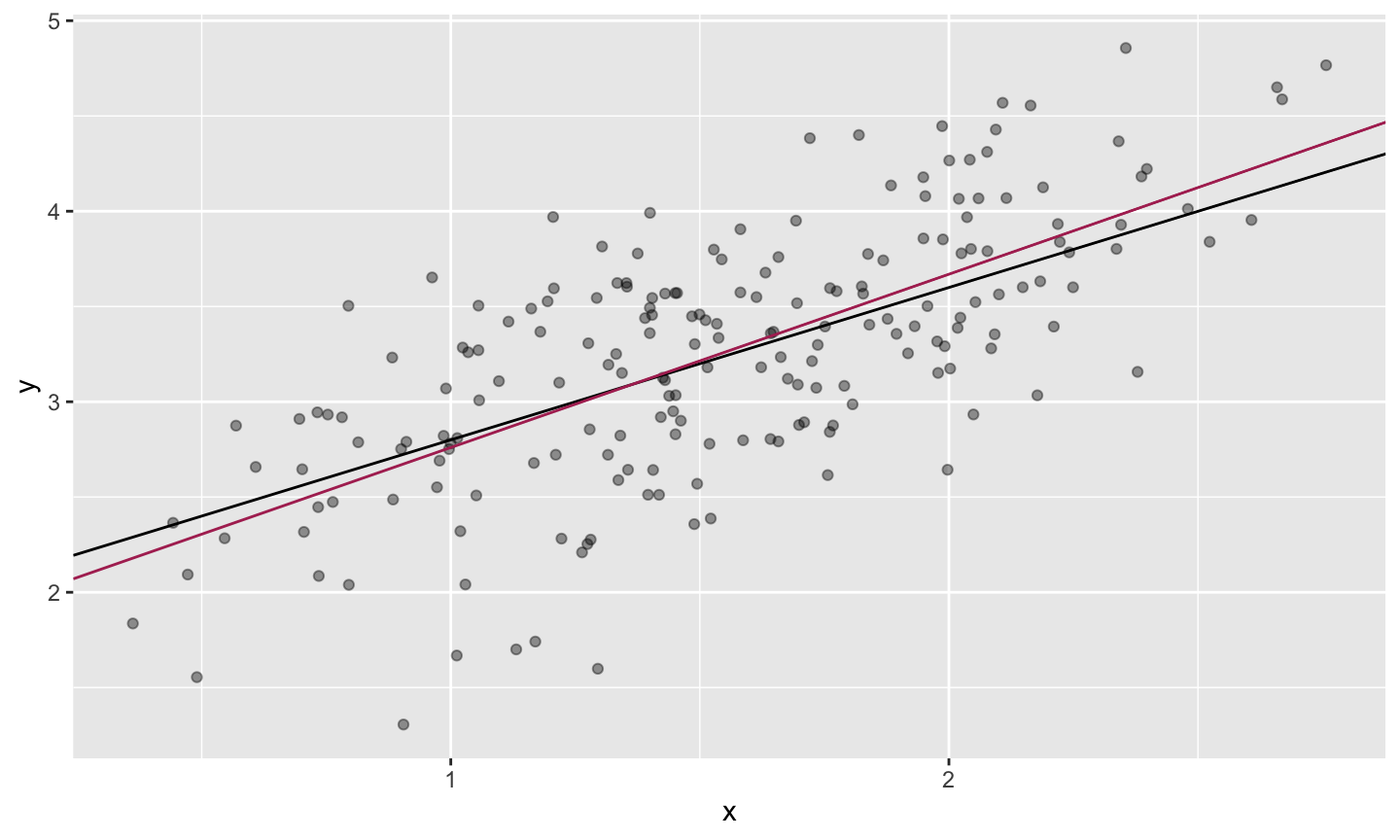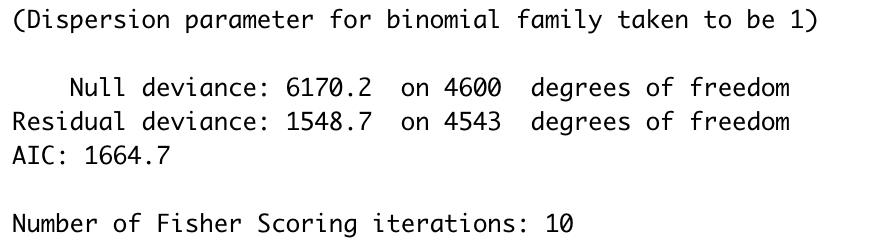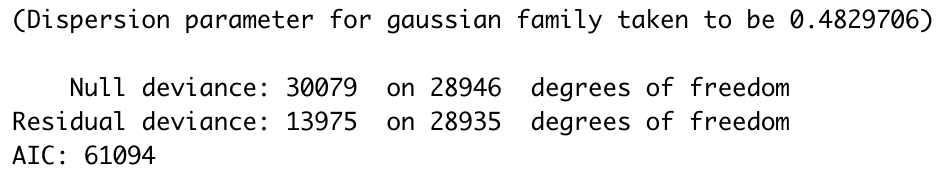April 8th, 2021

## Today’s Class

### Time permitting

1. Quick Review
• Linear Regression
• Logistic Regression
2. Deviance
3. Out-of-Sample performance
• Poisson, etc.
5. Inference
• Bootstrap?
6. Preview HW2

## Linear Regression

Many problems involve a response or outcome (y),
And a bunch of covariates or predictors (x) to be used for regression.

A general tactic is to deal in averages and lines.

$E[y|x] = f(x'\beta)$

Where $$x = [1,x_1,x_2,x_3,...,x_p]$$ is our vector of covariates. (Our number of covariates is $$p$$ again)
$$\beta = [\beta_0,\beta_1,\beta_2,...,\beta_p]$$ are the corresponding coefficients.
The product $$x'\beta = \beta_0+\beta_1 x_1 + \beta_2 x_2+\cdots+\beta_p x_p$$.

For simplicity we denote $$x_0 = 1$$ to estimate intercepts

## Example## Logistic Regression

Building a linear model for binary data.
Recall our original specification: $$E[Y|X] = f(x'\beta)$$

The Response $$y$$ is 0 or 1, leading to a conditional mean: $E[y|x] = P[y=1|x]\times 1 + P[y=0|x]\times 0 = P[y=1|x]$ $$\implies$$ The expectation is a probability.

The ‘logit’ link is common, for a few reasons. One big reason? $log\left(\frac{p}{1-p}\right) =\beta_0+\beta_1x_1+...+\beta_Kx_K$ This is a linear model for log odds.

## Deviance

Deviance refers to the distance between our fit and the data. You generally want to minimize it.

$Dev(\beta) = -2 log(L(\beta|data))+C$ We can ignore C for now.

Deviance is useful for comparing models. It is a measure of GOF that is similar to the residual sum of squares, for a broader class of models (logistic regression, etc).

We’ll think about deviance as a cost to be minimized.

Minimize Deviance $$\iff$$ Maximize likelihood

Bringing this full circle.

$\phi(x) = \frac{1}{\sqrt{2\pi}}exp\left(- {x^2 \over 2}\right)$ Given $$n$$ independent observations, the likelihood becomes: $\prod_{i=1}^n \phi\left(y-x'\beta \over \sigma \right) \propto \prod_{i=1}^n exp\left(-{(y-x'\beta)^2 \over 2 \sigma^2} \right)$ $\propto exp \left(-{1 \over 2\sigma^2} \sum_{i=1}^n (y-x'\beta)^2 \right)$

This leads to Deviance of: $Dev(\beta) = -2log(L(\beta|data)) + C = {1 \over \sigma^2} \sum_{i=1}^n (y-x'\beta)^2 + C'$

So:
Min Deviance $$\iff$$ Max likelihood $$\iff$$ Min $$l_2$$ loss

This is just a particular loss function, which is driven by the distribution of the $$\epsilon$$ terms.

## MLE for Logistic Regression

Our logistic regression has the following likelihood: $L(\beta) = \prod_{i=1}^n P[y_i|x_i] = \prod_{i=1}^n p_i^{y_i}(1-p_i)^{1-y_i}$ $= \prod_{i=1}^n \left({exp(x_i'\beta) \over 1+exp(x_i'\beta)}\right)^{y_i} \left({1 \over 1+exp(x_i'\beta)}\right)^{1-y_i}$ Thus the deviance to minimize is: $Dev(\beta) = -2 \sum_{i=1}^n (y_ilog(p_i)+(1-y_i)log(1-p_i))$ $\propto \sum_{i=1}^n [ log(1+exp(x_i'\beta))-y_ix_i'\beta]$

This is just taking the logs and removing the factor of 2.

Back to our summary outputs. We can print the same output for both linear and logistic regressions.

But the “dispersion parameter” is always 1 for the logistic regression. summary(spam)‘degrees of freedom’ is actually ‘number of observations - df’ where df is the number of coefficients estimated in the model.

Specifically df(deviance) = nobs - df(regression)

You should be able to back out number of observations from the R output.

## Dispersion parameter for Linear regression?

summary(reg.bse)Remember our basic gaussian model was: $Y|X \sim N(X'\beta,\sigma^2)$ And the implied deviance was: $Dev(\beta) = {1 \over \sigma^2}\sum_{i=1}^n (y-x'\beta)^2 +C'$

$$\sigma$$ is the dispersion parameter, and it is critical here. The logit has a mean-variance link, so we don’t need the separate param.

## Estimating $$\sigma$$

$y_i = x_i'\beta+\epsilon_i; \quad \sigma^2 = Var(\epsilon)$ Denote the residuals, $$r_i = y_i-x_i'\hat{\beta}$$.

$\hat{\sigma}^2 = {1 \over n-p-1} \sum_{i=1}^n r_i^2$

R calls $$\hat{\sigma}^2$$ the dispersion parameter.

Critically, even if we know $$\beta$$, we only predict sales with uncertainty.
E.g., approximately a 95% chance of sales in $$x'\beta \pm 2\sqrt{0.48}$$

## $$R^2$$

Residual Deviance, $$D$$ is what we’ve minimized using $$x$$.
Null Deviance $$D_0$$ is for the model without $$x$$ (or more generally, the model under the null).
i.e. $$\hat{y}_i = \bar{y}$$

• $$D_0 = \sum (y_i-\bar{y})^2$$ in linear regression
• $$D_0 = -2\sum[y_ilog(\bar{y})+(1-y_i)log(1-\bar{y})]$$ in logits

The difference between $$D$$ and $$D_0$$ comes from information in $$x$$.

Proportion of deviance explained by $$x$$ is called the $$R^2$$ in a linear regression, “Pseudo-$$R^2$$” in logit. $R^2 = {D_0-D \over D_0} = 1-{D \over D_0}$

This measures how much variability you explain with your model.

• In spam: $$R^2 = 1-1549/6170 = 0.75$$
• In OJ – reg.bse: $$R^2 = 1-13975/30079 = 0.54$$

## $$R^2$$ in linear regression

Recall that for linear model, deviance is the sum of squared errors (SSE) and $$D_0$$ is the total sum of squares (TSS). $R^2 = 1-{SSE \over TSS}$

You may also recall that $$R^2 = corr(y,\hat{y})^2$$.

cor(reg.bse$fitted,oj$logmove)^2
##  0.5353939

For linear regression, min deviance $$=$$ max corr$$(y,\hat{y})$$. $$\implies$$ if $$y$$ vs $$\hat{y}$$ is a straight line, you have a perfect fit.

Also implies that $$R^2$$ (weakly) increases whenever we add another variable.

## Fit plots

fitplotdf = data.frame(y = oj$logmove,yhat= predict(reg.bse),brand=oj$brand)
ggplot(fitplotdf,aes(y=y,x=yhat,col=brand)) +
geom_jitter(alpha=0.2,size=0.2,width=0.03)+
geom_abline(intercept=0,slope=1)